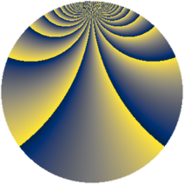# Properties

 Label 552.2.bfLevel $552$ Weight $2$ Character orbit 552.bf Rep. character $\chi_{552}(5,\cdot)$ Character field $\Q(\zeta_{22})$ Dimension $920$ Newform subspaces $1$ Sturm bound $192$ Trace bound $0$

# Related objects

## Defining parameters

 Level: $$N$$ $$=$$ $$552 = 2^{3} \cdot 3 \cdot 23$$ Weight: $$k$$ $$=$$ $$2$$ Character orbit: $$[\chi]$$ $$=$$ 552.bf (of order $$22$$ and degree $$10$$) Character conductor: $$\operatorname{cond}(\chi)$$ $$=$$ $$552$$ Character field: $$\Q(\zeta_{22})$$ Newform subspaces: $$1$$ Sturm bound: $$192$$ Trace bound: $$0$$

## Dimensions

The following table gives the dimensions of various subspaces of $$M_{2}(552, [\chi])$$.

Total New Old
Modular forms 1000 1000 0
Cusp forms 920 920 0
Eisenstein series 80 80 0

## Trace form

 $$920q - 22q^{4} - 2q^{6} - 44q^{7} - 18q^{9} + O(q^{10})$$ $$920q - 22q^{4} - 2q^{6} - 44q^{7} - 18q^{9} - 22q^{10} - 12q^{12} - 22q^{15} - 30q^{16} - 10q^{18} - 14q^{24} + 40q^{25} - 22q^{28} - 11q^{30} - 20q^{31} - 22q^{33} - 18q^{36} - 42q^{39} - 176q^{40} - 11q^{42} + 90q^{46} - 64q^{48} + 32q^{49} - 90q^{52} + 21q^{54} - 76q^{55} - 22q^{57} + 76q^{58} - 11q^{60} - 22q^{63} - 28q^{64} - 44q^{66} - 116q^{70} - 79q^{72} - 36q^{73} - 22q^{76} - 134q^{78} - 44q^{79} - 34q^{81} - 40q^{82} - 165q^{84} - 18q^{87} - 22q^{88} - 198q^{90} + 88q^{94} - 231q^{96} - 44q^{97} + O(q^{100})$$

## Decomposition of $$S_{2}^{\mathrm{new}}(552, [\chi])$$ into newform subspaces

Label Dim. $$A$$ Field CM Traces $q$-expansion
$$a_2$$ $$a_3$$ $$a_5$$ $$a_7$$
552.2.bf.a $$920$$ $$4.408$$ None $$0$$ $$0$$ $$0$$ $$-44$$